APPLIED INDUSTRIAL CONTROL SOLUTIONS LLCNormalized (per-normal or per-unit) Units in Control System Analysis

General

When an engineer is confronted with the task of designing control systems, one of two approaches will form the foundation of his analysis. The first approach, which at first glance seems the easiest, will involve using engineering units throughout the design, and then, at the time it becomes necessary to implement the design into a controller, convert the gains and frequencies into numbers that the PLC or DCS firmware understands. The second, and at first glance more difficult, approach is to normalize all the units and render the design unitless.

The main advantage to the second approach is that the size of the units used does not influence the intuitive feel that one is capable of acquiring when using this approach. For example the concept of per-normal inertia (J_Bar) states that this unitless quantity is equal to the time it takes in seconds to accelerate the given inertia to motor base speed with rated motor torque. If J_Bar is equal to 100 [sec] then we can say that the motor is "small" in terms of power, compared to the load, whether the load be a 100,000 [lb ft^2] drum, or a 1 [oz in^2] disk platter. We can say this because the motor takes a relatively long time to accelerate the load. Similarly If J_Bar is equal to 1 [msec] we can say that the motor is "large" in terms of power, because it can accelerate the load to top speed so quickly.

Similar observations can be made for all the normalized units presented below. The final advantage to using per-normal units is that the gains of the controller always mean the same thing. i.e. a gain of 60 in a speed loop is large, always in the same sense, despite the sizes of the motor or the load.

With the above in mind, the following tutorial is intended to provide some background material for the reader interested in understanding the ApICS approach to using per-unit solutions in systems applications. It is intended as reference material only. It contains some definitions of physical quantities, base quantities and normalized quantities that are used in standard ApICS stability analysis and application software.

Scope

All the definitions, contained in this appendix, apply to ApICS stability analysis and our standard approach to generating application software.

Conventions

Normalized Quantities

A normalized quantity is defined here as a physical quantity that is multiplied and/or divided by one or more base quantities. A physical quantity, when normalized, gains significance as a result of being expressed in terms of fixed base quantities.

Very often, a physical quantity is normalized, by dividing the physical quantity by a base quantity with the same engineering units as the physical quantity. As a result, the normalized quantity does not have units, but is ‘expressed in per-unit’. The normalized quantity has a value of 1.0 [per-unit] when the physical quantity has a value equal to the base quantity.

For stability analysis, it is often convenient to normalize a physical quantity, in a way that will give the normalized quantity units of seconds. For example, inertia can be normalized by multiplying by motor base speed and dividing by motor rated torque. The value of normalized inertia has units of seconds and represents the acceleration time to motor base speed with motor rated torque applied. Dancer position can be normalized by dividing by maximum line speed. The value of normalized position has units of seconds and represents the time to move the dancer from full position to empty position at maximum line speed.

Symbols

Symbols are used to represent physical quantities in mathematical equations. The selection of these symbols follows several guidelines.

The symbol is made up of one or more English or Greek characters, with an optional subscript and/or bar. A symbol, without a subscript or bar, represents an instantaneous physical quantity in engineering units. The subscript indicates a condition under which the quantity is fixed. For example, diameter has the symbol D and full roll diameter has the symbol DFR. A symbol with a bar indicates a normalized quantity that is expressed in per-unit. A symbol with a double bar indicates a normalized quantity that has units of seconds.

Drive

A drive is a combination of the power converter (controller), motor, and any motor mounted auxiliary devices.

Symbol Table

 Symbol Description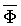Flux [Per Unit]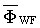Weak Field Flux [Per Unit] GR Gear Ratio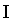Motor Current [A]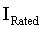Motor Rated Current [A]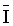Motor Current [Per Unit] J Reflected Inertia [Engineering units of choice]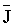Reflected Inertia [Per Unit]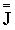Reflected Inertia [sec] Pwr Motor Rated (Nameplate) Power [Engineering units of choice] r Material Density [Engineering units of choice]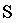Motor Speed [RPM] SNP Motor Rated (nameplate) Base Speed [RPM] SB Motor Base Speed [RPM] SMV Motor Maximum Voltage Speed [RPM] SWF Motor Rated (Nameplate) Weak-Field Speed [RPM]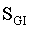Motor Gear-In Speed [RPM] SMSS Motor Maximum Safe Speed [RPM] SFinal Motor Final Speed [RPM]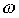Motor Angular Speed [rad/sec]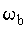Motor Base Angular Speed [rad/sec] wWF Motor Rated (Nameplate) Weak-Field Angular Speed [rad/sec]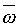Motor Angular Speed [Per Unit]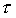Motor Torque [Engineering units of choice]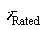Motor Rated Torque [Engineering units of choice]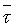Motor Torque [Per Unit]

Definitions

Motor Flux

Normalized Motor Flux ()

Normalized Motor Flux is defined as the ratio of instantaneous motor flux to rated motor flux.

Maxi-torque is a method of maximizing available motor torque by setting the motor field current equal to motor rated field current below motor base speed and as high as possible above base speed.

Two parameters may limit the maximum allowable field strength above base speed, rated motor armature voltage and inverting fault setback voltage. Inverting fault setback voltage is the maximum armature voltage that does not cause inverting faults. Inverting fault setback voltage, when required, is less than rated motor armature voltage.

Maxi-torque control can be implemented with an armature voltage regulator, a CEMF regulator, or a field current reference with a saturation curve.

With maxi-torque control, Normalized Motor Flux can be defined in terms of motor speed: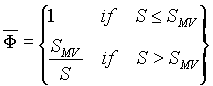Motor Current

Motor Current (I)

Motor current is defined as the average armature current for a DC motor or the fundamental RMS motor current for an AC motor. Motor current has units of [Amperes].

Motor Rated Current ()

Motor rated current is defined as the motor nameplate full load amperes.

Normalized Motor Current ()

Normalized motor current is defined as the ratio of instantaneous motor current to motor rated current: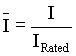Motor Speed

Motor Speed (S)

Motor Speed is the motor shaft rotation velocity in revolutions per minute [RPM].

Motor Rated (Nameplate) Base Speed (SNP)

Motor Base Speed in [RPM] as shown on the motor nameplate.

Motor Base Speed (SB)

The motor speed in [RPM] with rated voltage, rated field strength and rated load with the windings at the constant temperature attained when operated at its ratings. This speed may vary slightly from the Motor Rated Base Speed (SNP) found on the motor nameplate.

From Nema MG-1-1993 12.70

The variation above or below the rated full-field speed of a DC motor shall not exceed 7.5% when operated at rated load and voltage and at full field with the windings at the constant temperature attained when operating at its ratings.

Occasionally motors, especially AC motors, are rated at a voltage less than the maximum operating voltage. In this case, Motor Base Speed (SB) is less than the speed corresponding to maximum operating voltage, rated field strength and rated load. The application’s constant torque region is reduced and the application’s constant horsepower region is extended electronically.

In any case, if the exact Motor Base Speed (SB) is not known, Motor Base Speed (SB) can be set equal to the Motor Rated Base Speed (SNP) found on the motor nameplate.

Motor Maximum Voltage Speed (SMV)

The motor speed in [RPM] with maximum operating voltage, rated field strength and rated load with the windings at the constant temperature attained when operated at its ratings. Motor Maximum Voltage Speed (SMV) is typically equal to Motor Base Speed (SB).

Motor Rated (Nameplate) Weak-Field Speed (SWF)

Motor maximum operating speed in [RPM] as shown on the motor nameplate or motor electrical data.

Motor Gear-in Speed (SGI)

Motor maximum operating speed in [RPM] for a particular application.

Motor Maximum Safe Speed (SMSS)

Maximum safe operating speed of the motor [RPM]. This speed must not be exceeded under any conditions.

Motor Angular Speed ()

Motor Angular Speed is the motor shaft angular velocity in radians per second [rad/sec]. Motor Angular Speed [rad/sec] can be calculated from Motor Speed [RPM] as follows: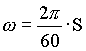Motor Base Angular Speed (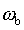)

Motor Base Angular Speed is the Motor Base Speed in radians per second [rad/sec]. Motor Base Angular Speed [rad/sec] can be calculated from Motor Base Speed [RPM] as follows: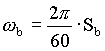Motor Rated Weak Field (Nameplate) Angular Speed (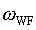)

Motor Rated Weak Field Angular Speed is the Motor Rated Weak Field Speed in radians per second [rad/sec]. Motor Rated Weak Field Angular Speed [rad/sec] can be calculated from Motor Rated Weak Field Speed [RPM] as follows: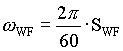Normalized Motor Angular Speed ()

Normalized Motor Angular Speed is defined as the ratio of instantaneous angular motor speed [rad/sec] to the motor rated angular speed [rad/sec]: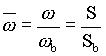Motor Torque

Motor Torque ()

Motor torque is defined as the instantaneous motor shaft torque.

Rated Motor Torque (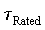)

Rated motor torque is the motor torque produced at motor base speed and motor rated power. Rated motor torque can be calculated as follows:

In Imperial units: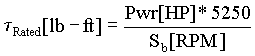In SI units: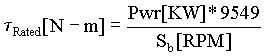Normalized Motor Torque ()

Normalized motor torque is defined as the ratio of instantaneous motor torque to motor rated torque: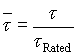Inertia

Reflected Inertia (J)

Reflected inertia is defined as the sum of all inertia’s (e.g. motor, gearbox, brake, sheaves, rolls) connected to the motor shaft after being properly reflected though any gear ratios.

Note: Some conversion factors of interest:

• Weight Density: 1 [lb/in3] = 1728 [lb/ft3]
• Mass Density: 1 [slug/ft3] = 0.5154 [g/cm3] ( 1 [g/cm3] = 1000 [kg/m3] )
• Length: 1 [ft] = 12 [in.] = 0.3048 [m] = 30.48 [cm] = 304.8 [mm]
• Force: 1 N = 0.2248 [lb]

Normalized Reflected Inertia [sec] ()

Normalized reflected inertia [sec] is the instantaneous inertia, normalized by multiplying by motor base speed and dividing by the motor rated torque. It is the time in seconds that it takes to accelerate the motor from zero speed to motor base speed with motor rated torque and the instantaneous reflected inertia. See Figure 2 for the determination of normalized reflected inertia [sec] from a current (torque) test. Normalized reflected inertia [sec] can be calculated as follows:

In Imperial units: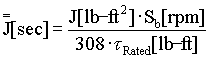In SI units: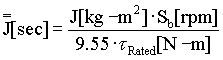Miscellaneous Equations and Unit Conversions

Torque

Normalized Torque Balance Equation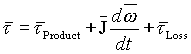where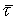is the motor torque,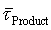is the normalized torque applied to the product, and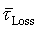is the normalized losses torque.